# Introduction to the Options Screener

The OpenBB options screener is a versatile discovery tool for an options trader. It is powered by the Open Source Community: https://github.com/Tyruiop/syncretism - go give this repository a star!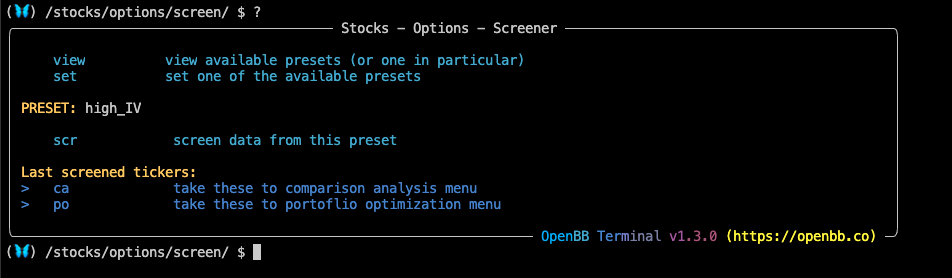The screener makes use of presets (.ini files) that are stored locally in the OpenBB installation folder.

`~/Applications/OpenBB/openbb_terminal/stocks/options/presets/`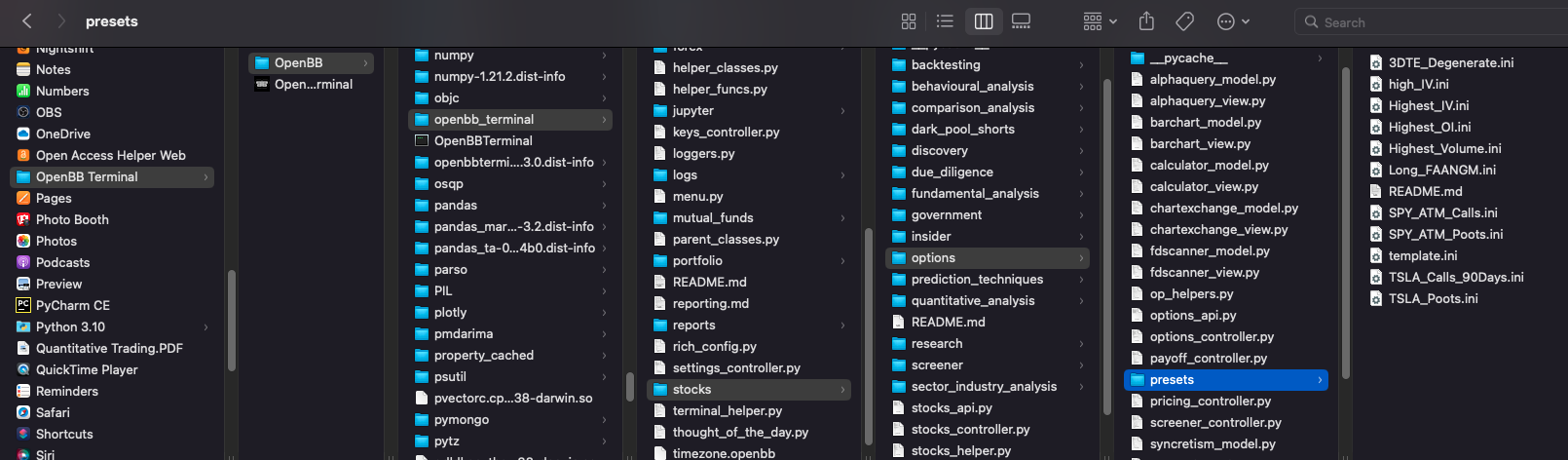The file named `template.ini` is a blank slate and ready for experimentation. There are nearly infinite ways to configure an options search, so customize one and then share it with us on social media! Have a look at the available fields:

``````# Author of preset: OpenBB Terminal
# Description: This is just a sample. The user that adds the preset can add a description for what type of stocks these filters are being used.
[FILTER]

# tickers [string]: a list of space or comma separated tickers to restrict or exclude them from the query.
# E.g. "GME" or for multiples "AMC","BB","GME".
tickers =

# exclude [true|false]: if true, then tickers are excluded. If false, the search is restricted to these tickers.
exclude =

# min-diff [int]: minimum difference in percentage between strike and stock price.
min-diff =

# max-diff [int]: maximum difference in percentage between strike and stock price.
max-diff =

# itm [bool]: select in the money options.
itm = true

# otm [bool]: select out of the money options.
otm = true

# min-exp [int]: minimum days until expiration.
min-exp =

# max-exp [int]: maximum days until expiration.
max-exp =

# min-price [float]: minimum option premium.
min-price =

# max-price [float]: maximum option premium.
max-price =

#min-strike [float]: minimum option strike.
min-strike =

#max-strike [float]: maximum option strike.
max-strike =

# calls [true|false]: select call options.
calls = true

# puts [true|false]: select put options.
puts = true

# stock [true|false]: select normal stocks.
stock =

# etf [true|false]: select etf options.
etf =

# min-sto [float]: minimum option price / stock price ratio.
min-sto =

# max-sto [float]: maximum option price / stock price ratio.
max-sto =

# min-yield [float]: minimum premium / strike price ratio.
min-yield =

# max-yield [float]: maximum premium / strike price ratio.
max-yield =

# min-myield [float]: minimum yield per month until expiration date.
min-myield =

# max-myield [float]: maximum yield per month until expiration date.
max-myield =

# min-delta [float]: minimum delta greek.
min-delta =

# max-delta [float]: maximum delta greek.
max-delta =

# min-gamma [float]: minimum gamma greek.
min-gamma =

# max-gamma [float]: maximum gamma greek.
max-gamma =

# min-theta [float]: minimum theta greek.
min-theta =

# max-theta [float]: maximum theta greek.
max-theta =

# min-vega [float]: minimum vega greek.
min-vega =

# max-vega [float]: maximum vega greek.
max-vega =

#min-iv [float]: minimum implied volatility.
min-iv =

#max-iv [float]: maximum implied volatility.
max-iv =

#min-oi [float]: minimum open interest.
min-oi =

#max-oi[float]: maximum open interest
max-oi =

#min-volume [float]: minimum volume.
min-volume =

#max-volume [float]: maximum volume.
max-volume =

#min-voi [float]: minimum volume / oi ratio.
min-voi =

#max-voi [float]: maximum volume / oi ratio.
max-voi =

# min-cap [float]: minimum market capitalization (in billions USD).
min-cap =

# max-cap [float]: maximum market capitalization (in billions USD).
max-cap =

# order-by [default: e_desc]: how to order results, possible values:
#     e_desc, e_asc: expiration, descending / ascending.
#     iv_desc, iv_asc: implied volatility, descending / ascending.
#     lp_desc, lp_asc: lastprice, descending / ascending.
#     md_desc, md_asc: current stock price, descending / ascending.
order-by =

# limit [int]: number of results (max 50).
limit =

# active [true|false]: if set to true, restricts to options for which volume, open interest, ask, and bid are all > 0.
active =

# [float|int] deviation from the 20 day average
min-price-20d =
max-price-20d =
min-volume-20d =
max-volume-20d =
min-iv-20d =
max-iv-20d =
min-delta-20d =
max-delta-20d =
min-gamma-20d =
max-gamma-20d =
min-theta-20d =
max-theta-20d =
min-vega-20d =
max-vega-20d =
min-rho-20d =
max-rho-20d =

# [float|int] deviation from the 100 day average
min-price-100d =
max-price-100d =
min-volume-100d =
max-volume-100d =
min-iv-100d =
max-iv-100d =
min-delta-100d =
max-delta-100d =
min-gamma-100d =
max-gamma-100d =
min-theta-100d =
max-theta-100d =
min-vega-100d =
max-vega-100d =
min-rho-100d =
max-rho-100d =
``````

## How to use the Options Screener

The commands `set` and `view` will show the filters present. The command reads the contents of the preset file every time the command is called, which makes changes fast and easy; simply open it in a text editor.

``````(🦋) /stocks/options/screen/ \$ set TSLA_Calls_90Days

(🦋) /stocks/options/screen/ \$ view TSLA_Calls_90Days

- FILTER -
tickers    : "TSLA"
exclude    : false
itm        : true
otm        : true
min-exp    : 3
max-exp    : 90
calls      : true
puts       : true
stock      : true
etf        : true
min-delta  : 0.30
max-delta  : 0.65
order-by   : "oi_desc"
active     : true
``````

The columns for the output of the screener have been abbreviated to be:

``````CS: Contract Symbol; S: Symbol, T: Option Type; Str: Strike; Exp v: Expiration; IV: Implied Volatility; LP: Last Price; B: Bid; A: Ask; V: Volume; OI: Open Interest; Y: Yield; MY: Monthly Yield; SMP: Regular Market Price; SMDL: Regular Market Day Low; SMDH: Regular Market Day High; LU: Last Trade Date; LC: Last Crawl; ITM: In The Money; PC: Price Change; PB: Price-to-book.
``````

The default number of results returned is 10, add the `-l` flag with the number of desired results to change.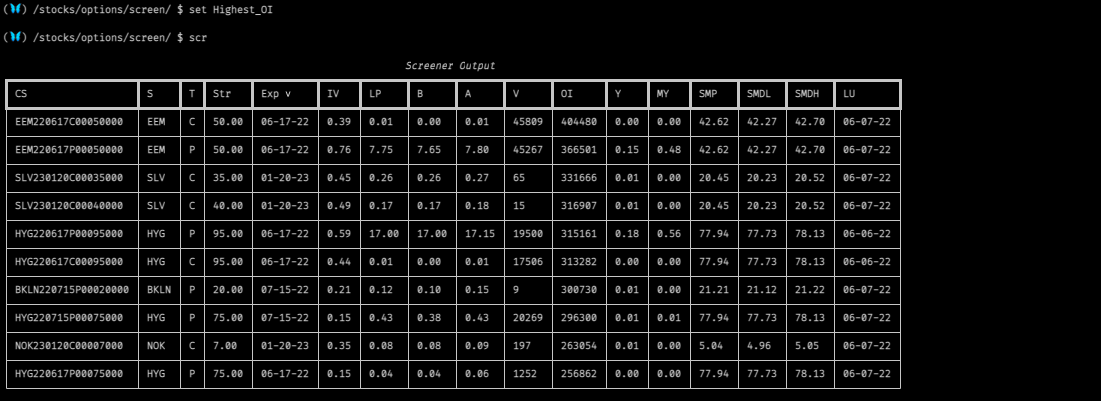## Examples

Using the preset, “3DTE_Degenerate”: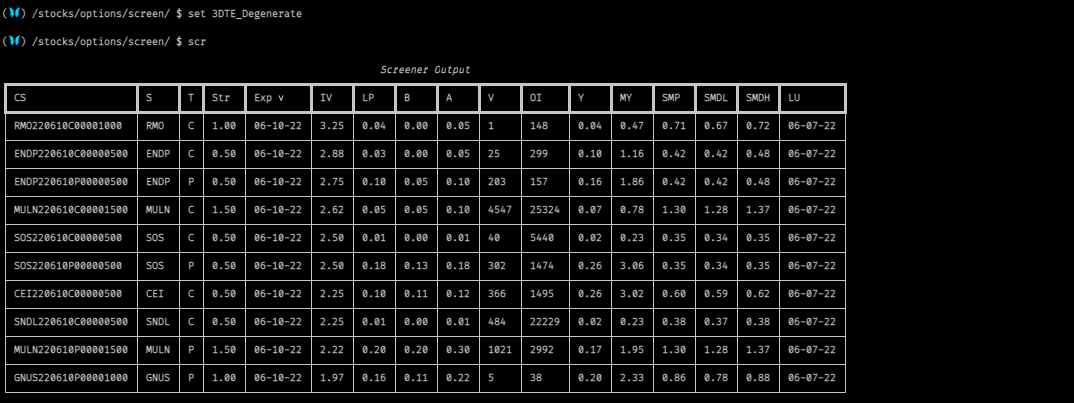Using the preset, “SPY_ATM_POOTS”: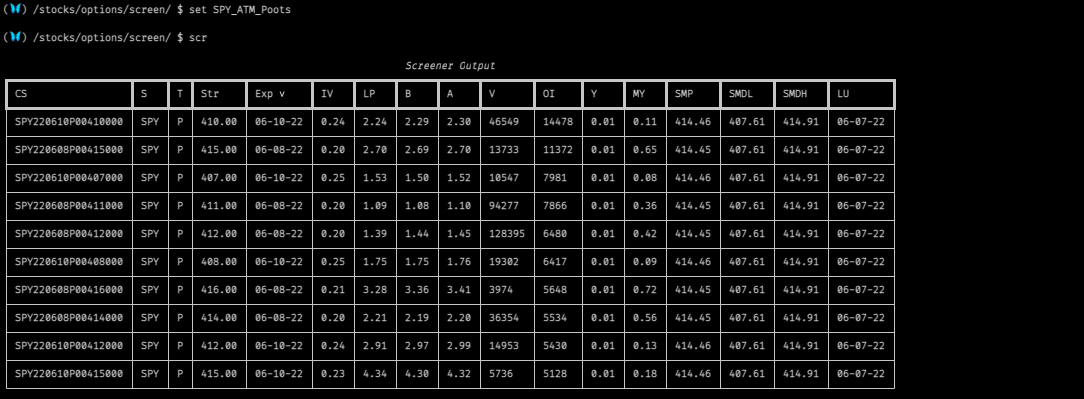The video below shows the process for building an options screener preset. Any simple text editor will work to make changes. Build a preset and share it with OpenBB on social media!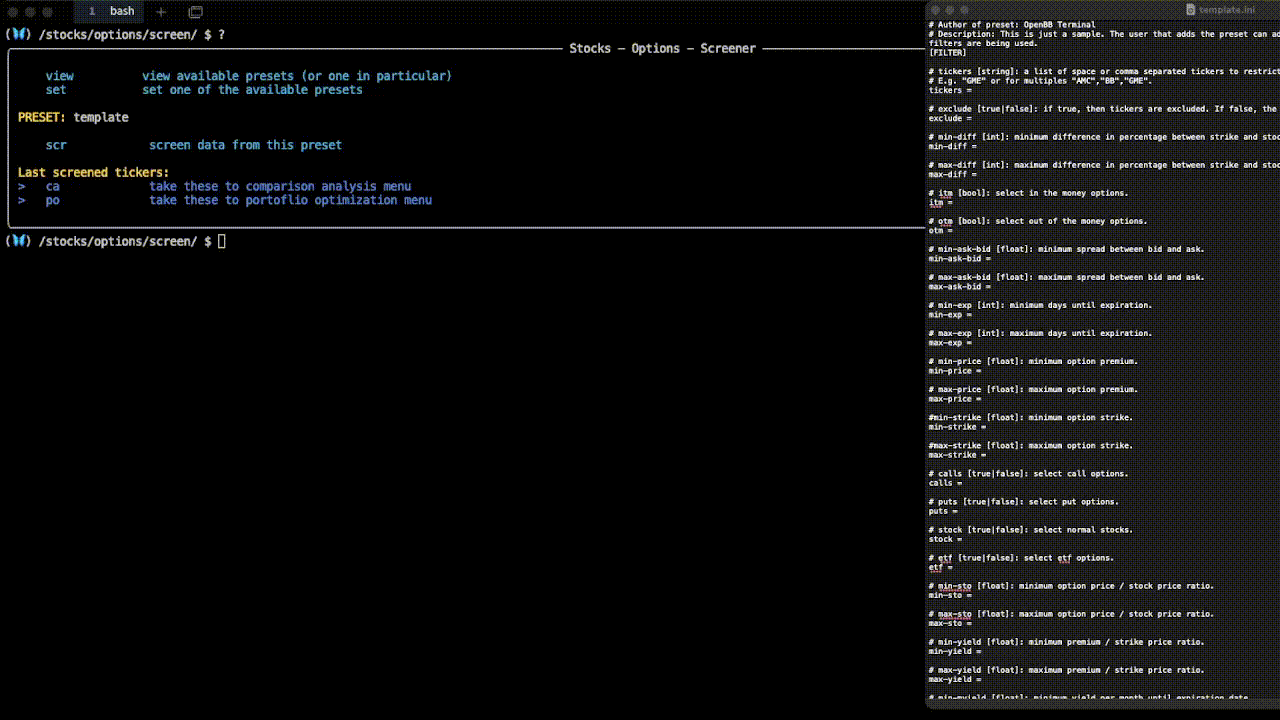Back to: Introduction To Options Guide